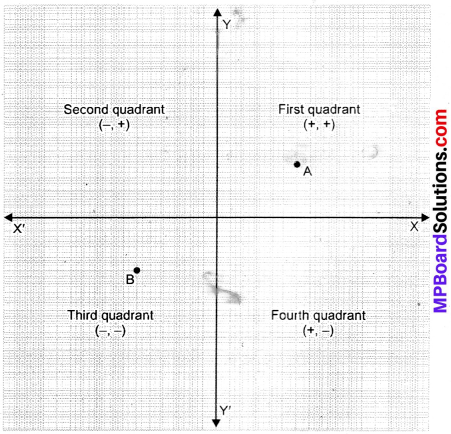# MP Board Class 9th Maths Solutions Chapter 3 Coordinate Geometry Ex 3.1

## MP Board Class 9th Maths Solutions Chapter 3 Coordinate Geometry Ex 3.1

Question 1.
How will you describe the position of a table lamp on your study table to another person?
Solution:
Consider the lamp as a point and table as a plane. Choose any two perpendicular edges of the table. Measure the distance of the lamp from the longer edge, suppose it is 25 cm. Again, measure the distance of the lamp from the shorter edge, and suppose it is 30 cm. You can write the position of the lamp as (30, 25) or (25, 30), depending on the order you fix.Question 2.
(Street Plan):
A city has two main roads which cross each other at the center of the city. These two roads are along the North – South direction and East – West direction. All the other streets of the city run parallel to these roads and are 200 m apart. There are 5 streets in each direction. Using 1 cm = 200 m, draw a model of the city on your notebook. Represent the roads/streets by single line.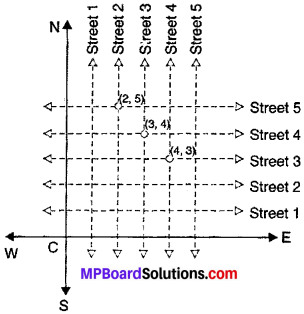There are many cross – streets in your model. A particular cross-street is made by two streets, one running in the North – South direction and another in the East – West direction. Each cross – street is referred to in the following, manner: If the 2nd street running in the North – South direction and 5th in the East – West direction meet at some crossing, then we will call this cross-street (2, 5). Using this convention, find:

1. How many cross-streets can be referred to as (4, 3)?
2. How many cross-streets can be referred to as (3, 4)?

Solution:
Both the cross-streets are marked in the figure given. They are uniquely found because of the two reference lines we have used for locating them.

Cartesian Coordinate Axes:
These are two mutually perpendicular reference lines shown in Fig. which locate a point is a plane.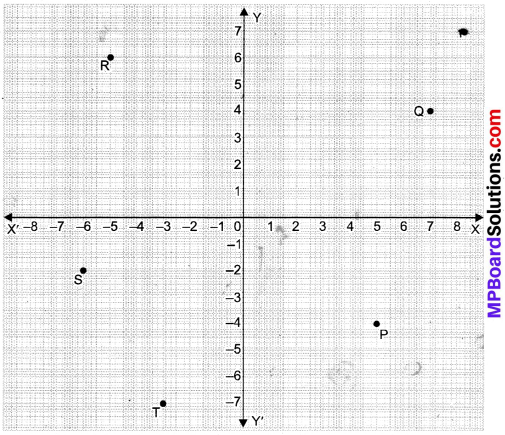Convention of Signs:
OX is the positive direction and OX’ is the negative direction of x – axis while OY is positive and OY’ is negative.
In other words,

On the X – axis:
Values to the right are positive and values to the left are negative.

On the Y – axis:
Values above are positive and those below are negative.

Cartesian System:
The system used for describing the position of a point in a plane is called the Cartesian system.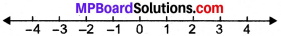Origin:
The point at which the two coordinate axes meet is called the origin. when a point is in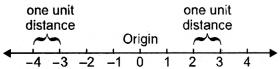I. Quadrant: Y – value + and Y – value +
II. Quadrant: Y – value – and Y – value +
III. Quadrant: Y – value – and Y – value –
IV. Quadrant: Y – value + and Y – value –

The X – coordinate of a point is perpendicular distance from Y – axis, is known as abscissa. The Y – coordinate of a point is known as ordinate is perpendicular distance from Y – axis.